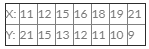Courses

# Test: Correlation And Regression- 5

## 40 Questions MCQ Test Business Mathematics and Logical Reasoning & Statistics | Test: Correlation And Regression- 5

Description
This mock test of Test: Correlation And Regression- 5 for CA Foundation helps you for every CA Foundation entrance exam. This contains 40 Multiple Choice Questions for CA Foundation Test: Correlation And Regression- 5 (mcq) to study with solutions a complete question bank. The solved questions answers in this Test: Correlation And Regression- 5 quiz give you a good mix of easy questions and tough questions. CA Foundation students definitely take this Test: Correlation And Regression- 5 exercise for a better result in the exam. You can find other Test: Correlation And Regression- 5 extra questions, long questions & short questions for CA Foundation on EduRev as well by searching above.
QUESTION: 1

Solution:
QUESTION: 2

Solution:
QUESTION: 3

### Since the correlation coefficient r cannot be greater than 1 numerically, the product of the regression must

Solution:
QUESTION: 4

The correlation coefficient r is the __________ of the two regression coefficients byx and bxy

Solution:
QUESTION: 5

Which are is true

Solution:
QUESTION: 6

Maximum value of Rank Correlation coefficient is

Solution:
QUESTION: 7

The partial correlation coefficient lies between

Solution:
QUESTION: 8

r12 is the correlation coefficient between

Solution:
QUESTION: 9

r12 is the same as r21

Solution:
QUESTION: 10

In case ‘Age and income’ correlation is

Solution:
QUESTION: 11

In case ‘Speed of an automobile and the distance required to stop the car often applying brakes’ – correlation is

Solution:
QUESTION: 12

In case ‘Sale of woolen garments and day temperature’–––– correlation is

Solution:
QUESTION: 13

In case ‘Sale of cold drinks and day temperature’ –––––– correlation is

Solution:
QUESTION: 14

In case of ‘Production and price per unit’ – correlation is

Solution:
QUESTION: 15

If slopes at two regression lines are equal them r is equal to

Solution:
QUESTION: 16

Co–variance measures the joint variations of two variables.

Solution:
QUESTION: 17

The minimum value of correlation coefficient is

Solution:
QUESTION: 18

The maximum value of correlation coefficient is

Solution:
QUESTION: 19

When r = 0 , the regression coefficients are

Solution:
QUESTION: 20

For the regression equation of Y on X , 2x + 3Y + 50 = 0. The value of bYX is

Solution:
QUESTION: 21

In Method of Concurrent Deviations, only the directions of change (Positive direction / Negative direction) in the variables are taken into account for calculation of

Solution:
QUESTION: 22

In calculating the Karl Pearson’s coefficient of correlation it is necessary that the data should be of numerical measurements. The statement is

Solution:
QUESTION: 23

Correlation coefficient can be found out by

Solution:
QUESTION: 24

In rank correlation coefficient the association need not be linear

Solution:
QUESTION: 25

Great advantage of _________ is that it can be used to rank attributes which can not be expressed by way of numerical value.

Solution:
QUESTION: 26

Correlation coefficient is ___________ of the units of measurement.

Solution:
QUESTION: 27

Rank correlation coefficient lies between

Solution:
QUESTION: 28

A coefficient near +1 indicates tendency for the larger values of one variable to be associates with the larger values of the other

Solution:
QUESTION: 29

Correlation methods are used to study the relationship between two time series of data which are recorded annually, monthly, weekly, daily and so on.

Solution:
QUESTION: 30

The value of correlation coefficient lies between

Solution:
QUESTION: 31

In rank correlation coefficient only an increasing/decreasing relationship is required

Solution:
QUESTION: 32

Correlation coefficient is a pure number

Solution:
QUESTION: 33

Age of Applicants for life insurance and the premium of insurance – correlations are

Solution:
QUESTION: 34

The sum of the difference of rank is

Solution:
QUESTION: 35

Karl Pearson’s coefficient is defined from

Solution:
QUESTION: 36

Covariance measures __________ variations of two variables.

Solution:
QUESTION: 37

For y = 25, what is the estimated value of x, from the following data:Solution:
QUESTION: 38

Correlation coefficient between two variables is a measure of their linear relationship

Solution:
QUESTION: 39

The following data relate to the heights of 10 pairs of fathers and sons:
(175, 173), (172, 172), (167, 171), (168, 171), (172, 173), (171, 170), (174, 173), (176, 175), (169, 170), (170, 173)
The regression equations of height of son on that of father is given by

Solution:
QUESTION: 40

When high values of one variable are associated with high values of the other & low values of one variable are associated with low values of another, then they are said to be

Solution: